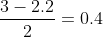Scan QR code or get instant email to install app

Question:

# Books Forever, Inc., uses short-term bank debt to buy inventory. Assuming an initial current ratio that is greater than 1, and an initial quick (or acid test) ratio that is less than 1, what is the effect of these transactions on the current ratio and the quick ratio?

A Both ratios will decrease.
explanation

1212As an example, start with CA = 2, CL = 1, and Inv = 1.2. We begin with a current ratio of 2 and a quick ratio of 0.8. If the firm increases short-term bank debt (a current liability) by 1 to buy inventory (a current asset) of 1, both the numerator and denominator increase by 1, resulting in 3/2 =1.5 (new current ratio) and